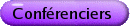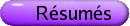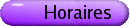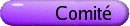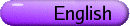Équations différentielles et systèmes dynamiques
Org: Elena Braverman (Calgary) et Michael Y. Li (Alberta)
[PDF]

Relative Dynamics in Systems Biology
[PDF]

The aim of this work is to design a method for developing mathematical models in order to apply on natural dynamical systems. Discussions on biological knowledge, in terms of objects, concepts and rules, suggest a need for mathematical models and novel methodologies to contribute to the conceptual or theoretical framework in studying dynamics on organisms. Through this paper, we will describe our mathematical method, "observational modelling", which is based on system theory, factor-space theory, dynamical systems and fuzzy logic to provide a relational description of dynamic on genomic systems, metabolic systems and systems biology. In the other part, we are going to apply Schopenhauer's philosophy to the specific dynamical systems, "relative dynamical systems", through observational modelling. In these kind of systems the Rule Bases could have their own dynamic, or some how modification. However, investigating the conflict between this dynamic and original dynamic of system is the aim.

BARUCH CAHLON, Oakland University, Dept. of Math., Rochester, MI 48309, USA
Stability Criteria for Certain High Even Order Delay Differential Equations
[PDF]

In this paper we study the asymptotic stability of the zero solution of even order linear delay differential equations of the form

 y(2m)(t) = 2m-1å j=0 aj y(j)(t) + 2m-1å j=0 bjy(j) (t-t)
where aj and bj are certain constants and m ³ 1. Here t > 0 is a constant delay. In proving our results we make use of Pontryagin's theory for quasi-polynomials.

It is clear that with 4m independent parameters in (1.1) one cannot expect to get regions of stability. Our goal is to derive algorithmic type stability criteria.

ABBA GUMEL, University of Manitoba
Dynamics Analysis of HIV Vaccine Models
[PDF]

Since its emergence in the 1980s, the human immunodeficiency syndrome (HIV) continues to inflict major public health and socio-economic burdens globally. Currently, 34-46 million people live with HIV and over 20 million have so far died of the disease. Although the use of anti-retroviral therapy (ART) has been quite effective in slowing HIV spread in some nations, it is generally believed that the global control of the HIV pandemic would require a vaccine. Thankfully, a number of candidate vaccines are currently undergoing various stages of clinical trials ... and there is a need to qualitatively analyse their potential impact. This talk will focus on designing and analysing HIV vaccine models, which incorporate some of the key biological features of HIV and expected vaccine characteristics, to assess the potential impact of an imperfect vaccine in combatting the HIV pandemic.

HONGBIN GUO, University of Alberta
Global Dynamics of Multigroup SIR Epidemic Models
[PDF]

For a class of multigroup SIR epidemic models with varying subpopulation sizes, the global dynamics are completely determined by the basic reproduction number R0. More specifically, if R0 £ 1, then the disease-free equilibrium is globally asymptotically stable; if R0 > 1, then there exists a unique endemic equilibrium and it is globally asymptotically stable in the interior of the feasible region.

SHAFIQUL ISLAM, University of Lethbridge
Piecewise linear approximation of absolutely continuous invariant measures for random maps
[PDF]

Fixed points of Frobenious-Perron Operators for random maps are the probability density functions of absolutely continuous invariant measures for the random maps. The Frobenious-Perron equation is a functional equation and solving this equation explicitly is possible only in very simple cases. In this talk we describe a method of approximating fixed point of the Frobenious-Perron Operator for random maps. We prove the convergence of our piecewise linear approximation method.

DAMIR KINZEBULATOV, University of Calgary, 2500 University Drive NW, Calgary, Alberta T2N 1N4
On Nicholson's blowflies equation with a distributed delay
[PDF]

The dynamics of the well-known Nicholson's blowflies model,

 N¢(t) = pN(t-t) eaN(t-t) - dN(t), \tag1
(1)
where N(t) is the size of the population at time t, coefficients p, d ³ 0 are the maximal daily egg production and adult death rates, respectively, 1/a is the population size providing maximal reproduction rate and t ³ 0 is the generation time, was intensively studied in the literature in the last decades.

It is believed that models with a distributed delay (generally, time dependent) provide a more adequate description of population dynamics than equations with a constant concentrated delay. In this talk, we consider the Nicholson's blowflies model with a time-dependent distributed delay. Global and local behaviour of solutions is investigated: positiveness and persistence, global attractivity and oscillation.

BILL LANGFORD, University of Guelph, Ontario
Models of Cheyne-Stokes Respiration with Cardiovascular Pathologies
[PDF]

Cheyne-Stokes respiration is a periodic breathing pattern, characterized by short intervals of deep breathing each followed by an interval of very little or no breathing (known as apnea). This work improves on previous compartmental models of the human cardio-respiratory system that simulate concentration of carbon dioxide in compartments of the cardiovascular system and the lungs. The parameter boundary on which Hopf bifurcation gives birth to a period oscillation has been determined. The models predict that an increase in either the ventilation-perfusion ratio or feedback gain can give rise to stable Cheyne-Stokes oscillations. Physiologically, it is observed that Cheyne-Stokes respiration is more likely to occur in people with pathologies such as chronic heart failure or encephalitis, or in healthy humans during acclimatization to high altitudes or after hyperventilation. Modifications of the model to incorporate these conditions give good agreement with the observations.

This paper is joint with Fang Dong.

RONGSONG LIU, York University
Spatiotemporal Patterns of Vector-borne Disease Spread
[PDF]

There are many factors contributing to the complicated and interesting spatiotemporal spread patterns of vector-borne diseases. Here, we focus on two major factors: the demographic and disease ages and the spatial movement of the disease hosts. We derive from a structured population model a system of delay differential equations describing the interaction of five sub-populations for a vector-born disease with particular reference to West Nile virus, and we also incorporate the spatial movements by considering the analogue reaction-diffusion systems with non-local delayed terms. Specific conditions for the disease eradication and sharp conditions for the local stability of the disease-free equilibrium are obtained using comparison techniques coupled with the spectral theory of monotone linear semiflows. A formal calculation of the minimal wave speed for the traveling waves is given.

JAMES MULDOWNEY, University of Alberta, Edmonton
Implications of the stability of linearizations
[PDF]

Let x = f(t) be a bounded solution of the C1 autonomous system x¢ = f(x) in Rn. It is an exercise to show that the omega limit set of this solution is a stable hyperbolic equilibrium if and only if the linearized system y¢ = [(f)/(x)] ( f(t) ) y is uniformly asymptotically stable. This talk will present similar conditions for the omega limit set to be a stable hyperbolic equilibrium or a homoclinic or heteroclinic cycle with certain attraction properties.

Work is joint with Michael Li.

GERGELEY RÖST, York University, Toronto / Univ. Szeged, Hungary
Bifurcation of periodic delay differential equations
[PDF]

We study the bifurcation of time-periodic scalar delay differential equations, depending on a parameter. The complete bifurcation analysis is performed explicitly, using Floquet-multipliers, spectral projection and center manifold reduction. The case of strong resonance is also discussed. Numerous examples are given to illustrate our theorems: subcritical and supercritical bifurcations into invariant tori can be observed for many notable equations.

SAMIR SAKER, Calgary
Periodic solutions, oscillation and attractivity of nonlinear delay discrete survival red blood cells model
[PDF]

In this paper, we will consider the discrete nonlinear delay survival red blood cells model

 x(n+1) - x(n) = -(n)x(n) + p(n) exp æè -q(n) xm(n-) öø ,    n = 1,2,...,
where (n), p(n) and q(n) are positive sequences of period and m is a positive constant. By using the continuation theorem in conincidence degree theory as well as some priori estimates we prove that the equation has a positive periodic solution x(n). We prove that the solutions are permanence and establish some sufficent conditions for the prevalence of the survival cells around the periodic solution which are oscillation criteria of the positive solutions about x(n). Also, we give an estimation of the lower and uper bounds of the osillatory solution and establishe some sufficient conditions for for the nonexistence of dynamical diseases on the population which are the global attractivity results of x(n).

PAULINE VAN DEN DRIESSCHE, University of Victoria, Victoria, BC
Modeling relapse in infectious diseases
[PDF]

An integro-differential equation is proposed to model a general relapse phenomenon in infectious diseases including herpes. The basic reproduction number R0 for the model is identified and the threshold property of R0 established. For the case of a constant relapse period (giving a delay-differential equation), this is achieved by conducting a linear stability analysis, and employing the Lyapunov-Razumikhin technique and monotone dynamical systems theory for global results. Numerical simulations, with parameter values relevant for herpes, are presented to complement the theoretical results, and no evidence of oscillatory solutions is found.

Joint work with Xingfu Zou.

QIAN WANG, University of Alberta
Some Results On A Diffusive SIR Model
[PDF]

The model we are interested in is given by

 St
 = d1 Sxx + L - b(x) IS - b1 S,
 It
 = d2 Ixx + b(x) IS - (b2 + g) I,
 0 < x < 1,  t > 0
 Rt
 = d3 Rxx + gI - b3 R,
with homogeneous Neumann boundary conditions, where S, I, R denote the number of susceptible, infectious and recovered population, respectively. In this talk, I will present some results on the existence and the structure of global attractor, and the existence of positive steady states. We prove that there exists an threshold parameter R0, whose sign determines the structure of the global attractor. If R0 £ 0, the disease-free equilibrium P0 is globally stable. If R0 > 0, P0 is unstable, and a positive, x-dependent endemic equilibrium P* exists. In the special case when b(x) = constant, P* is independent of x and is globally stable.

This is a joint work with Michael Y. Li.

YUAN YUAN, Memorial University of Newfoundland
Synchronization and Desynchronization in a Delayed Discrete Neural Network
[PDF]

We consider a delayed discrete neural network of two identical neurons with excitory interactions. After investigating the stability of the given system, we establish a new scheme and use the scheme to analyze the possible bifurcations occurring in the model. The process from its stable equilibrium to its multiple periodic patterns is explored clearly. A clarification for the asymptotically synchronous/asynchronous regions of such a system with Z2 symmetry is included.

ZINGFU ZOU, University of Western Ontario
Rich dynamics in a non-local population model over three patches
[PDF]

We consider a system of nonlinear delay differential equations that describes the mature population of a species with age-structure that lives over three patches. We analyze existence of nonnegative homogeneous equilibria, stability and Hopf bifurcation from the equilibria. In particular, by employing both the standard Hopf bifurcation theory and the symmetric bifurcation theory of functional differential equations, we obtain very rich dynamics for the system, such as transient oscillations, phase-locked oscillations, mirror-reflecting waves and standing waves. In the standard Hopf bifurcation case, we also derive formulas for determining the stability and the direction of the Hopf bifurcation.